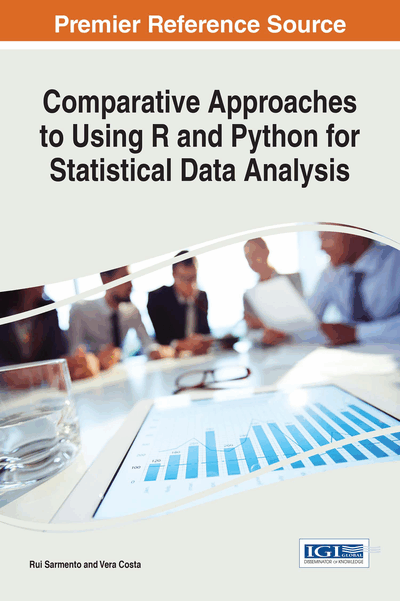# Introduction to Linear Regression

Copyright: © 2017 |Pages: 8
DOI: 10.4018/978-1-68318-016-6.ch006
OnDemand PDF Download:
Available
\$37.50
No Current Special Offers

## Abstract

In statistical modelling, regression analysis is a statistical process for estimating the relationships among variables. More specifically, regression analysis helps the reader understand how the dependent variable changes when any of the independent variables is varied. Thus, regression analysis estimates the average value of the dependent variable when the independent variables are fixed. Therefore, the estimation target is a function of the independent variables called regression function. In limited circumstances, regression analysis can be used to infer causal relationships between the independent and dependent variables. Nonetheless, caution has to be taken since correlation might not signify causality. Regression analysis techniques are varied. Nevertheless, in this chapter, we will present only the fundamental analysis.
Chapter Preview
Top

## R Vs. Python

To make a linear regression model, the variability of Publications variable was studied when related with the Gender, R_user, Python_user, and Age variables.

### Linear Regression Model

In linear regression model, the functional relationship between the dependent variable and the independent variablesare (Maroco, 2011):

Considering that the population is not defined, the linear regression analysis should start with the estimation of the regression coefficients from a representative sample of the population under study, using the estimators

producing sample estimatesof the population's parameters. The commonly used methods for estimation of these coefficients are mostly very laborious. Most software has extensive modules for linear regression. Thus, they eliminate the task of estimating these parameters; its detailed presentation will not be available in this book.

## Complete Chapter List

Search this Book:
Reset